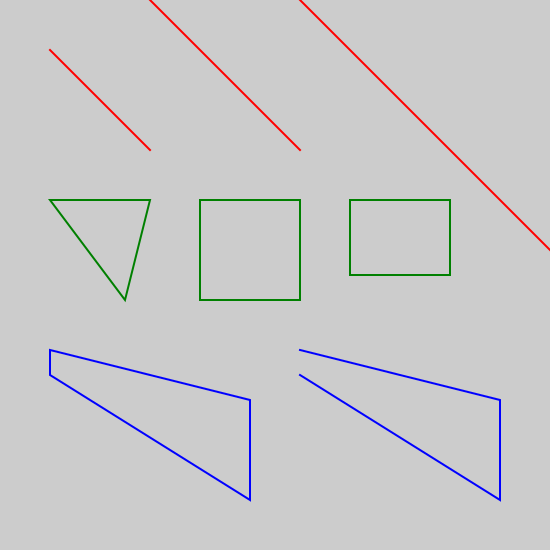# Polygons in generativepy

Martin McBride, 2020-10-07
Tags generativepy tutorial fill stroke rectangle square polygon triangle line
Categories generativepy generativepy tutorialThis tutorial shows how to create polygons in generativepy, using the geometry module. This includes the following shapes:

• Lines (segments, rays, and full lines)
• Triangles
• Squares
• Rectangles
• Polygons (open and closed)

For simplicity, the shapes are drawn as outlines. See the fill and stroke tutorial for details of how to fill and stroke shapes with different styles, and the patterns tutorial to learn about special fills such as gradients.

## Polygon example code

Here is the code to create various shapes:

from generativepy.drawing import make_image, setup
from generativepy.color import Color
from generativepy.geometry import Rectangle, Square, Triangle, Polygon, Line

def draw(ctx, pixel_width, pixel_height, frame_no, frame_count):

setup(ctx, pixel_width, pixel_height, background=Color(0.8))

red = Color('red')
green = Color('green')
blue = Color('blue')
thickness = 2

Line(ctx).of_start_end((150, 150), (50, 50)).stroke(red, thickness)
Line(ctx).of_start_end((300, 150), (200, 50)).as_ray().stroke(red, thickness)
Line(ctx).of_start_end((450, 150), (350, 50)).as_line().stroke(red, thickness)

Triangle(ctx).of_corners((50, 200), (150, 200), (125, 300)).stroke(green, thickness)
Square(ctx).of_corner_size((200, 200), 100).stroke(green, thickness)
Rectangle(ctx).of_corner_size((350, 200), 100, 75).stroke(green, thickness)

Polygon(ctx).of_points([(50, 350), (250, 400), (250, 500), (50, 375)]).stroke(blue, thickness)
Polygon(ctx).of_points([(300, 350), (500, 400), (500, 500), (300, 375)]).open().stroke(blue, thickness)

make_image("polygons-tutorial.png", draw, 550, 550)


This code is available on github in tutorial/shapes/polygons.py.

Here is the resulting image:## Lines

These lines, from the code above, draw the three red lines at the top of the image:

Line(ctx).of_start_end((150, 150), (50, 50)).stroke(red, thickness)
Line(ctx).of_start_end((300, 150), (200, 50)).as_ray().stroke(red, thickness)
Line(ctx).of_start_end((450, 150), (350, 50)).as_line().stroke(red, thickness)


The first Line defines a line from start point (150, 150) to end point (50, 50). It strokes the line using a red colour and a thickness of 2. This is the short line at the top left of the image. By default, a line goes from the start point to the end point but doesn't extend beyond those points. In mathematical terms, this is called a line segment.

The second Line adds an extra function as_ray. This creates a ray, sometimes called half-line. The line starts at (300, 150), passes through the point (200, 50), and then carries on forever. It is infinitely long in one direction.

The third Line adds an extra function as_line. This creates a full line. The line passes through the points (450, 150) and (350, 50), but it carries on forever in each direction. It is infinitely long in both directions.

## Named shapes

For convenience, there are several shape drawing objects, illustrated by these parts of the code above:

Triangle(ctx).of_corners((50, 200), (150, 200), (125, 300)).stroke(green, thickness)
Square(ctx).of_corner_size((200, 200), 100).stroke(green, thickness)
Rectangle(ctx).of_corner_size((350, 200), 100, 75).stroke(green, thickness)


Triangle has an of_corners method that accepts three points.

Square has an of_corner_size method that accepts a point and a side length.

Rectangle has an of_corner_size method that accepts a point, a width and a height.

Each of these shapes is stroked with a green line. They appear across the middle of the image.

## Polygons

The Polygon object can draw any polygon shape:

Polygon(ctx).of_points([(50, 350), (250, 400), (250, 500), (50, 375)]).stroke(blue, thickness)
Polygon(ctx).of_points([(300, 350), (500, 400), (500, 500), (300, 375)]).open().stroke(blue, thickness)


The of_points method accepts a sequence of points that represent the corners of the polygon. By default, the polygon will be drawn by joining all the points in order and joining the last point back to the first.

In the first Polygon, there are 4 points so the polygon has 4 sides. This is the green polygon at the bottom left of the image.

The second Polygon adds a call to the open method. This leaves the polygon open, in other words, it does not join the last point back to the first point. The polygon has 4 corners but only 3 sides, the other side is left open. This is the green polygon at the bottom right of the image.

If you found this article useful, you might be interested in the book Computer Graphics in Python or other books by the same author.

#### Popular tags

2d arrays abstract data type alignment and angle animation arange arc array arrays behavioural pattern bezier curve built-in function callable object chain circle classes clipping close closure cmyk colour combinations comparison operator comprehension context context manager conversion count creational pattern data science data types design pattern device space dictionary drawing duck typing efficiency ellipse else encryption enumerate fill filter font font style for loop function function composition function plot functools game development generativepy tutorial generator geometry gif global variable gradient greyscale higher order function hsl html image image processing imagesurface immutable object in operator index inner function input installing iter iterable iterator itertools join l system lambda function len line linear gradient linspace list list comprehension logical operator lru_cache magic method mandelbrot mandelbrot set map matplotlib monad mutability named parameter numeric python numpy object open operator optimisation optional parameter or pandas partial application path pattern permutations polygon positional parameter print pure function python standard library radial gradient range recipes rectangle recursion reduce repeat rgb rotation roundrect scaling scipy sector segment sequence setup shape singleton slice slicing sound spirograph sprite square str stream string stroke structural pattern subpath symmetric encryption template text text metrics tinkerbell fractal transform translation transparency triangle truthy value tuple turtle unpacking user space vectorisation webserver website while loop zip